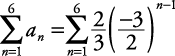## Summation Notation

A simple method for indicating the sum of a finite (ending) number of terms in a sequence is the summation notation. This involves the Greek letter sigma, Σ. When using the sigma notation, the variable defined below the Σ is called the index of summation. The lower number is the lower limit of the index (the term where the summation starts), and the upper number is the upper limit of the summation (the term where the summation ends). Consider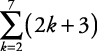This is read as “the summation of (2 k + 3) as k goes from 2 to 7.” The replacements for the index are always consecutive integers.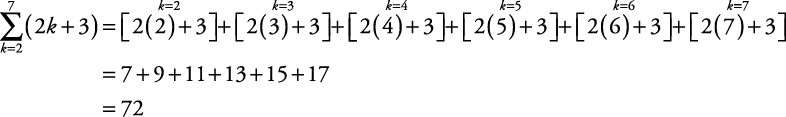##### Example 1

Write out the terms of the following sums; then compute the sum.

1.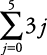2.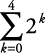3.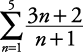1.2.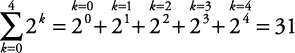3.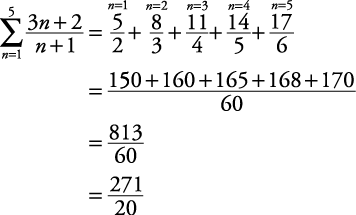##### Example 2

Use sigma notation to express each series.

1. 8 + 11 + 14 + 17 + 20

2.1. 8 + 11 + 14 + 17 + 20

This is an arithmetic series with five terms whose first term is 8 and whose common difference is 3. Therefore, a 1 = 8 and d = 3. The nth term of the corresponding sequence is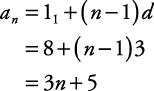Since there are five terms, the given series can be written as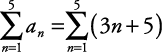2.This is a geometric series with six terms whose first term is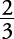and whose common ratio is. Therefore,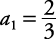and. The nth term of the corresponding sequence isSince there are six terms in the given series, the sum can be written as